You have no items in your shopping cart.
Search

# 1" of Water Application Time

Use this form to calculate the irrigation time to apply one inch of water with a center pivot. Find the irrigated area underneath the center pivot by using the pivot acreage calculator.

## 1" of Water Application Time

Area:
Flow Rate:
Percent of Full Circle:
%
Irrigation Efficiency:
%
Time:

### The Equation

This calculator uses this formula to determine the 1" application time.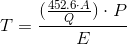Where: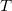= Time it takes to complete a cycle while applying 1" (hrs)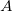= Irrigated Area (acres)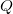= Flow rate at the pivot (gpm)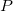= Percent of full circle being irrigated (as a decimal)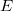= Irrigation efficiency (as a decimal)

Reference: Washington State University